# Bessel inequality

Jump to: navigation, search

The inequality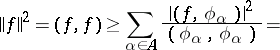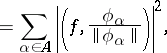whereis an element of a (pre-) Hilbert space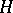with scalar productand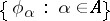is an orthogonal system of non-zero elements of. The right-hand side of Bessel's inequality never contains more than a countable set of non-zero components, whatever the cardinality of the index set. Bessel's inequality follows from the Bessel identity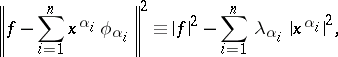which is valid for any finite system of elements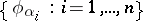. In this formula theare the Fourier coefficients of the vectorwith respect to the orthogonal system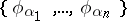, i.e.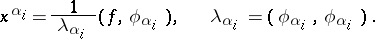The geometric meaning of Bessel's inequality is that the orthogonal projection of an elementon the linear span of the elements,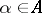, has a norm which does not exceed the norm of(i.e. the hypothenuse in a right-angled triangle is not shorter than one of the other sides). For a vectorto belong to the closed linear span of the vectors,, it is necessary and sufficient that Bessel's inequality becomes an equality. If this occurs for any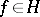, one says that the Parseval equality holds for the systemin.

For a system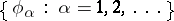of linearly independent (not necessarily orthogonal) elements ofBessel's identity and Bessel's inequality assume the form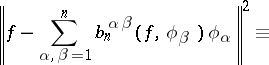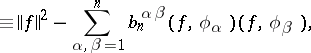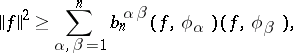where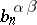are the elements of the matrix inverse to the Gram matrix (cf. Gram determinant) of the firstvectors of the initial system.

The inequality was derived by F.W. Bessel in 1828 for the trigonometric system.